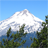# ODT termination CY7C2665KV18Level 1
Level 1

Hi,

I'm using tha sRAM CY7C2665KV18 and I'm using VDDQ = 1.8 V with Vref = 0.9 V.

1) Is the RQ calculation the same when VDDQ = 1.5 V?

In the "PROGRAMMABLE IMPEDANCE" section, the impedance is RQ*5.

In the "ON-DIE TERMINATION" section, the impedance range is 52.5 and 105 that follows RQ/3.3 for 175<= RQ <= 350 (ODT pin LOW).

2) What is the correct formula to calculate output impedance?

Thanks

Best regards

Bryan

1 Solution

# Re: ODT termination CY7C2665KV18ModeratorModeratorHi Bryan,

RQ resistor connected on ZQ pin is used for 2 purposes in the device CY7C2665KV18.

1. Configure On Die Termination (ODT) resistance value.

ODT resistance value is determined by the ODT pin.

"A low on this pin selects a low range that follows RQ/3.33 for 175Ohm <= RQ <= 350Ohm (where RQ is the resistor tied to ZQ pin). A HIGH on this pin selects a high range that follows RQ/1.66 for 175Ohm <= RQ <= 250Ohm(where RQ is the resistor tied to ZQ pin). When left floating, a high range termination value is selected by default."

2. Configure output impedance with which the SRAM drives data out.

Output impedance with with the SRAM driver data out will be RQ/5.

So, you have to choose an appropriate value for RQ resistor to configure both ODT resistance and output impedance of our device.

e.g. If a 250Ohm resistor is selected for RQ and ODT pin is LOW. Then.

ODT resistance = RQ/3.3 = 75.75Ohm

Output impedance = RQ/5 = 50Ohm

Thanks and Regards,

Sudheesh

3 Replies

# Re: ODT termination CY7C2665KV18ModeratorModeratorHi Bryan,

RQ resistor connected on ZQ pin is used for 2 purposes in the device CY7C2665KV18.

1. Configure On Die Termination (ODT) resistance value.

ODT resistance value is determined by the ODT pin.

"A low on this pin selects a low range that follows RQ/3.33 for 175Ohm <= RQ <= 350Ohm (where RQ is the resistor tied to ZQ pin). A HIGH on this pin selects a high range that follows RQ/1.66 for 175Ohm <= RQ <= 250Ohm(where RQ is the resistor tied to ZQ pin). When left floating, a high range termination value is selected by default."

2. Configure output impedance with which the SRAM drives data out.

Output impedance with with the SRAM driver data out will be RQ/5.

So, you have to choose an appropriate value for RQ resistor to configure both ODT resistance and output impedance of our device.

e.g. If a 250Ohm resistor is selected for RQ and ODT pin is LOW. Then.

ODT resistance = RQ/3.3 = 75.75Ohm

Output impedance = RQ/5 = 50Ohm

Thanks and Regards,

Sudheesh

# Re: ODT termination CY7C2665KV18Level 1
Level 1

In the example with RQ = 250 Ohm, the impedance of the tracks must be 50 Ohm, is it correct?

thanks

Bryan

# Re: ODT termination CY7C2665KV18ModeratorModeratorHi Bryan,

Yes, it is correct.

Thanks and Regards,

Sudheesh